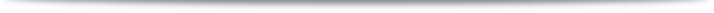﻿ KIAS Workshop on Combinatorics
 KIAS Combinatorics Workshop Series1st Workshop Home > 1st Workshop

The 1st KIAS Combinatorics Workshop was held at KIAS on November 8- 9, 2013.

• Date: November 8 - 9 (Fri-Sat), 2013

• Venue: Room 1503, KIAS

• Invited Speakers
Woong Kook (Seoul National University)
Sejeong Bang (Yeungnam University)
Heesung Shin (Inha University)

• List of Participants
• Schedule
[1st Day, November 8th]

14:00 ~ 14:50  <Talk 1>  Woong Kook
•                   "Simplicial Tree Numbers for Matroid Complexes"
15:00 ~ 15:50  <Talk 2>
Sejeong Bang
•                    "Spectral characterization of distance-regular graphs
•                      with the smallest eigenvalue \$theta_3geq-3\$"
Coffee Break
16:20 ~ 17:10  <Talk 3> Heesung Shin
•                     "Eulerian polynomials via continued fractions"
17:20 ~ 18:00   Problem session
18:00 ~ 20:00   Banquet
•  [2nd Day, November 9th]
•        09:00 ~ 10:00   Breakfast
•       10:00 ~ 12:00   Discussions

• Abstracts
• <Talk 1>  Woong Kook
•  "Simplicial Tree Numbers for Matroid Complexes"
In this talk we will review the definition of high-dimensional trees and show how weighted tree numbers are computed via combinatorial Laplacians \$Delta_{i}\$. This result is a generalization of Temperley's tree number formula for graphs (1964) to arbitrary acyclic complexes. As an application, we will discuss tree numbers of matroid complexes. The talk will begin with a brief survey of applications of \$Delta_{i}\$ to networks and topological data analysis.

• <Talk 2>  Sejeong Bang
• "Spectral characterization of distance-regular graphs  with the smallest eigenvalue \$theta_3geq-3\$"
Any connected graph has smallest eigenvalue at most \$-1\$ with equality if and only if the graph is complete. For connected regular graphs with smallest eigenvalue at least \$-2\$, it was shown by P. J. Cameron et al. that either it is a line graph, a cocktail party graph, or the number of vertices is at most \$28\$. Let \$Gamma\$ be a non-complete connected graph with diameter \$D\$. Then \$Gamma\$ is called {em distance-regular} if the \$i\$-th distance matrix \$A_i\$ can be expressed as a polynomial of degree \$i\$ in \$A_1\$, \$i=0,1,ldots, D\$. In this talk, we consider distance-regular graphs with diameter three and smallest eigenvalue at least \$-3\$.

• <Talk 3> Heesung Shin
• "Eulerian polynomials via continued fractions"
There are several bijections between permutations and Laguerre histories by Foata-Zeilberger, Françon-Viennot, and so on. These bijections give continued fraction formulas as a generating function for some statistics of permutations.

Using continued fraction expansion, it is shown that the sequence of coefficients of Eulerian polynomial is symmetric and unimodal. Recently, Blanco and Petersen (arXiv:1206.0803v2) conjectured a \$q\$-analogue of Eulerian polynomial as an expansion formula for inversions and excedances in the symmetric group. In this talk, we prove this conjecture.

Also, we can find a similar expansion for the eulerian polynomials of fixed-point free permutations of type B. This formula gives an answer to a conjecture of Mongelli (J. Combin. Theory Ser. A 120 (2013), no. 6, 1216–1234) about the unimodality of coefficients in the corresponding Eulerian polynomials.

• Problem Session
We hope to share and discuss interesting open problems in this session. If you are interested in proposing problems, please send an email to one of organizers before the workshop.